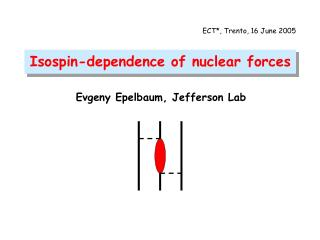# Isospin-dependence of nuclear forces - PowerPoint PPT PresentationDownload PresentationIsospin-dependence of nuclear forces

Download Presentation## Isospin-dependence of nuclear forces

- - - - - - - - - - - - - - - - - - - - - - - - - - - E N D - - - - - - - - - - - - - - - - - - - - - - - - - - -
##### Presentation Transcript

1. ECT*, Trento, 16 June 2005 Isospin-dependence of nuclear forces Evgeny Epelbaum, Jefferson Lab

2. Isospin structure of the 2N and 3N forces • Isospin-breaking nuclear forces in chiral EFT: • Two nucleons • Three nucleons • Summary and outlook Outline

3. Class II (charge independence breaking): charge reflection Class III (charge symmetry breaking, no isospin mixing): Class IV (charge symmetry breaking and isospin mixing): Isospin structure of the 2N force (Henley & Miller 1979) Class I (isospin invariant forces):

4. Generalization to 3 nucleons is not suitable for generalization to Conservation of since, in general: but Class I (isospin invariant forces): Class II (charge symmetry conserving): Class III (charge symmetry breaking):

5. Vertices: isospin invariant isospin breaking Chiral EFT à la Weinberg Unified expansion: N of nucleons N of loops N of vertices of type i van Kolck ’93, ‘95 Friar et al. ’03, ’04, … N of nucleon fields N of powers of the small scale

6. Class I > Class II > Class III > Class IV van Kolck ’93, ’95 (This hierarchy is valid for the specified power counting rules and assuming ). Hierarchy of the two-nucleon forces Class IV Class II Class III Class I Q0 Q1 Q2 Q3 Q4 Q5 + pure electromagnetic interactions (V1γ, V2γ, …)

7. Long-range electromagnetic forces • Dominated by the Coulomb interaction, vacuum polarization and the magnetic moment interaction (Ueling ’35, Durand III ’57, Stoks & de Swart ’90). • Contribute to Classes I, II, III, IV. • Big effects in low-energy scattering due to long range. πγ- exchange • Worked out by van Kolck et al., ‘98. • Contributes to Class II NN force at order Q4 . • Numerically small (α/π-times weaker than the isospin-invariant V1π). Isospin-violating contact terms • Up to orderQ5contribute to 1S0 and P-waves (Classes III, IV): 1S0 P-waves, CSB P-waves, spin & isospin mixing

8. Isospin-violating 1π-exchange potential Class IV potential: where (the NN Hamiltonian is still Galilean invariant, see Friar et al. ’04.) van Kolck ’93, ‘95; van Kolck, Friar & Goldman ’96; Friar et al. ’04; E.E. & Meißner ‘05 Q2 Q4 Q3 Class II Class III Classes II, III Class II Class IV (isospin mixing) Charge-dependent πNN coupling constant: [largely unknown…]

9. (Friar & van Kolck ’99): Trick CSB potential (non-polynomial pieces): Niskanen ’02; Friar et al. ’03, ’04; E.E. & Meißner ‘05. and where Class III Isospin-violating 2π-exchange potential: order Q4 take isospin-symmetric potential, , and use and: for pp and nn for np, T=1 Class II for np, T=0

10. CSBpotential (E.E. & Meißner ’05) where Class III and Isospin-violating 2π-exchange potential: order Q5 The CIB potential can be obtained using the above trick Class II

11. In the numerical estimation we use: • dimensional regularization, r [fm] GL ’82: • charge independentπN coupling, i.e.: . CSB2π-exchange potential: size estimation Subleading 2π-exchange potential is proportional to LECs c1, c3 and c4 which are large expect large contribution to the potential at order Q5

12. work in progress… Notice that formally: Class I > Class III > Class II Hierarchy of the three-nucleon forces (in an energy-independent formulation) Class II Class III Class I Q3 Q4 Q5

13. 1/m suppressed Feynman graphs=iteration of the NN potential (in an E-independent formulation) yield nonvanishing 3NF proportional to yields nonvanishing 3NF proportional to All 3NFs at Q4 are charge-symmetry breaking! 3N force: order Q4 (E.E., Meißner & Palomar ’04; Friar, Payne & van Kolck ‘04) Class II Class III Class III

14. Lead to nonvanishing 3NFs proportional to 3N force: order Q5 3N force: order Q5 (E.E., Meißner & Palomar ’04) Class II Leads to nonvanishing 3NF proportional to , Classes II, III Other diagrams lead to vanishing 3NF contributions: Feynman graphs=iteration of the NN potential 1/m suppressed

15. (!) • The strength of the Class II 3NFs: The formally subleading Class II 3NF is strong due to large values of ’s Size estimation(very rough) • The strength of the Class III 3NFs:

16. Role of the Δ EFT with explicit Δ’s would probably lead to the nuclear force contributions of a more natural size, since the big portion of the terms is shifted to lower orders. Δ-less EFT EFT with explicit Δ Q5 Q4 Q4 Q5

17. Summary • Isospin breaking nuclear forces have been studied up to order Q5. • 2N force • First contribute at order Q2. • Up to Q5, is given by 1γ-, 2γ-, πγ-, 1π-, 2π-exchange & contact terms. • Subleading (i.e. order- Q5) 2π-exchange numerically large! • The only unknown LECs in the long-range part are the charge dependent πNN coupling constants. They can [in principle] be fixed in PWA. • 3N force • First contribute at order Q4. • Depends on and the unknown LEC . • Numerically large CS-conserving force. Outlook • Numerical calculations in few-nucleon systems should be performed in order to see how large the effects actually are.